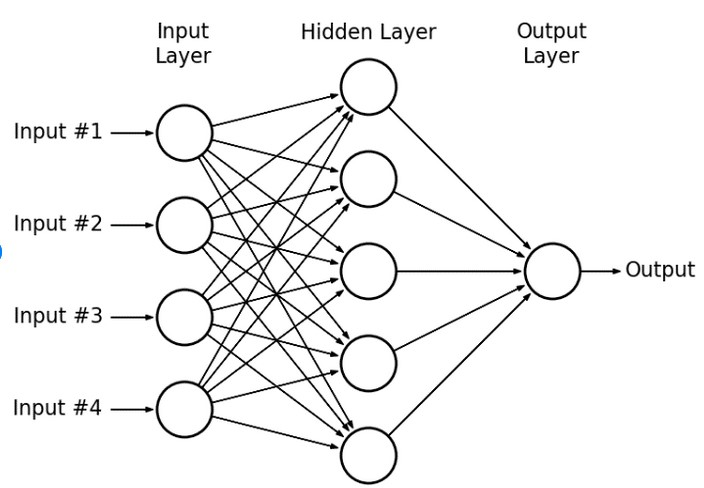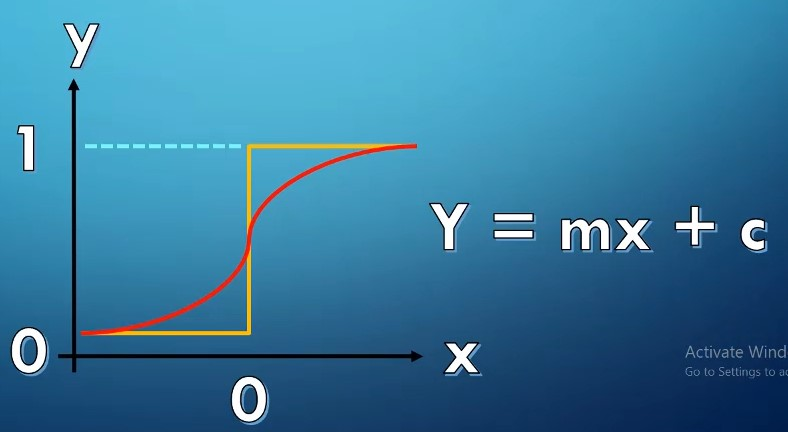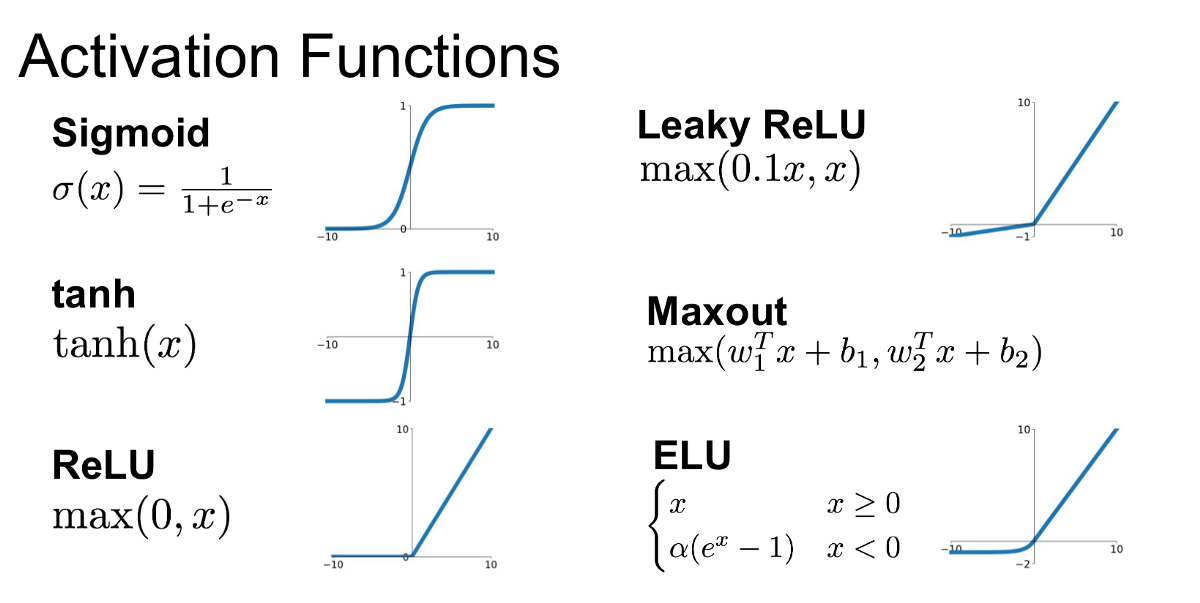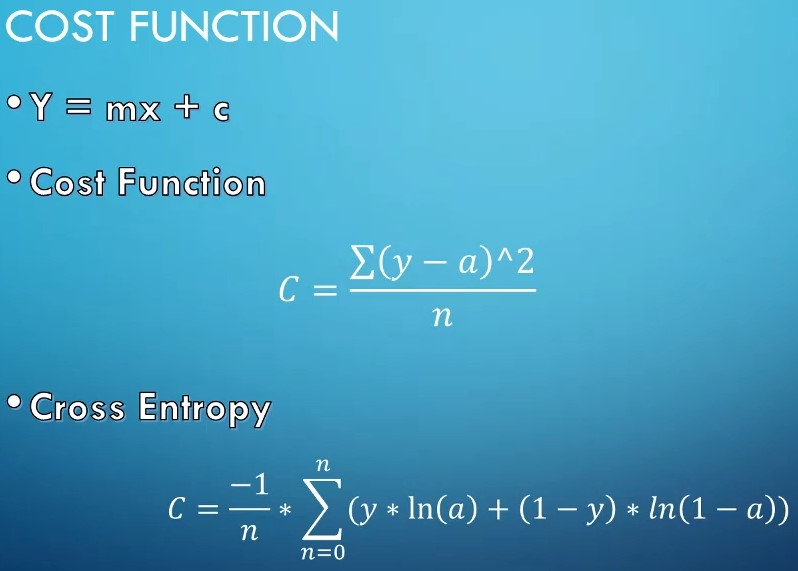# New Technology

Trending Technology Machine Learning, Artificial Intelligent, Block Chain, IoT, DevOps, Data Science## Search This Blog

IDEA of Neural Network :

We have already seen that how a single perceptron behaves, how let's explore this concept to the idea of a neural networks.

Now, let's how to connect many neurons (Perceptrons) together and then how to represent it mathematically.

Multiple Rerceptrons Network :-There are three layer -

1 INPUT LAYER :-

• Actual values of dataset

2. HIDDEN LAYERS :-

• These are layers in between inputs and outputs.
• If you have 3 or more layers then it will be Deep Neural Network.

3. Output Layer :-

• It contains the final output.

As you go forward through more layers, the level of abstraction increases.

Now let's discuss the activation function in a little more detail.

ACTIVATION FUNCTION:-

Previously our activation function was just a simple function that output 0 and 1.It would be nice if we could have a more dynamic function for example.Rectified Linear Unit (ReLU) - This is the most useful and relatively simple function, max(0,z).